Euler Equation

 |   Tiled Menu   |   Tables of Content   |  Banner Video   |  Tohline Home Page   |

Lagrangian Representation

in terms of velocity:

Among the principal governing equations we have included the

Lagrangian Representation
of the Euler Equation,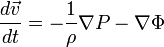$\frac{d\vec{v}}{dt} = - \frac{1}{\rho} \nabla P - \nabla \Phi$

[BLRY07], p. 13, Eq. (1.55)

in terms of momentum density:

Multiplying this equation through by the mass density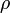$~\rho$ produces the relation,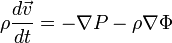$\rho\frac{d\vec{v}}{dt} = - \nabla P - \rho\nabla \Phi$ ,

which may be rewritten as,$\frac{d(\rho\vec{v})}{dt}- \vec{v}\frac{d\rho}{dt} = - \nabla P - \rho\nabla \Phi$ .

Combining this with the Standard Lagrangian Representation of the Continuity Equation, we derive,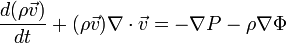$\frac{d(\rho\vec{v})}{dt}+ (\rho\vec{v})\nabla\cdot\vec{v} = - \nabla P - \rho\nabla \Phi$ .

Eulerian Representation

in terms of velocity:

By replacing the so-called Lagrangian (or "material") time derivative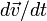$d\vec{v}/dt$ in the Lagrangian representation of the Euler equation by its Eulerian counterpart (see, for example, the wikipedia discussion titled, "Material_derivative", to understand how the Lagrangian and Eulerian descriptions of fluid motion differ from one another conceptually as well as how to mathematically transform from one description to the other), we directly obtain the

Eulerian Representation
of the Euler Equation,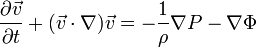$~\frac{\partial\vec{v}}{\partial t} + (\vec{v}\cdot \nabla) \vec{v}= - \frac{1}{\rho} \nabla P - \nabla \Phi$

in terms of momentum density:

As was done above in the context of the Lagrangian representation of the Euler equation, we can multiply this expression through by$~\rho$ and combine it with the continuity equation to derive what is commonly referred to as the,

Conservative Form
of the Euler Equation,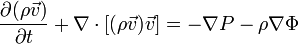$~\frac{\partial(\rho\vec{v})}{\partial t} + \nabla\cdot [(\rho\vec{v})\vec{v}]= - \nabla P - \rho \nabla \Phi$

[BLRY07], p. 8, Eq. (1.31)

The second term on the left-hand-side of this last expression represents the divergence of the "dyadic product" or "outer product" of the vector momentum density and the velocity vector, and is sometimes written as,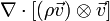$~\nabla\cdot [(\rho \vec{v}) \otimes \vec{v}]$.

in terms of the vorticity:

Drawing on one of the standard dot product rule vector identities, the nonlinear term on the left-hand-side of the Eulerian representation of the Euler equation can be rewritten as,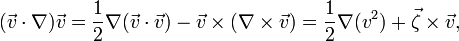$(\vec{v}\cdot\nabla)\vec{v} = \frac{1}{2}\nabla(\vec{v}\cdot\vec{v}) - \vec{v}\times(\nabla\times\vec{v}) = \frac{1}{2}\nabla(v^2) + \vec{\zeta}\times \vec{v} ,$

where,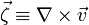$\vec\zeta \equiv \nabla\times\vec{v}$

is commonly referred to as the vorticity. Making this substitution leads to an expression for the,

Euler Equation
in terms of the Vorticity,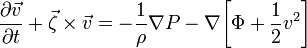$~\frac{\partial\vec{v}}{\partial t} + \vec\zeta \times \vec{v}= - \frac{1}{\rho} \nabla P - \nabla \biggl[\Phi + \frac{1}{2}v^2 \biggr]$

Double Check Vector Identities

In a subsection of an accompanying chapter titled, Double Check Vector Identities, we explicitly demonstrate for four separate "simple rotation profiles" that these two separate terms involving a nonlinear velocity expression do indeed generate identical mathematical relations, namely.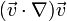$~(\vec{v} \cdot \nabla) \vec{v}$$~=$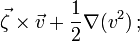$~\vec\zeta \times \vec{v} + \frac{1}{2}\nabla (v^2) \, ;$

and we explicitly demonstrate that they are among the set of velocity profiles that can also be expressed in terms of the gradient of a "centrifugal potential,"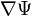$~\nabla\Psi$.

Related Discussions

 © 2014 - 2019 by Joel E. Tohline |   H_Book Home   |   YouTube   | Context: | PGE | SR | Appendices: | Equations | Variables | References | Binary Polytropes | Ramblings | Images | Images (2016 Layout) | ADS |